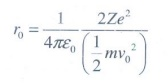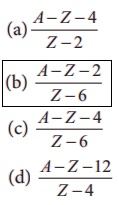Home | | Physics 12th Std | Multiple Choice Questions

# Multiple Choice Questions

Physics : Atomic and Nuclear Physics: Book Back Choose the correct Answers with Solution, Multiple choice questions with Answers and Solution: Multiple Choice Questions

I Multiple Choice Questions

1. Suppose an alpha particle accelerated by a potential of V volt is allowed to collide with a nucleus whose atomic number is Z, then the distance of closest approach of alpha particle to the nucleus is

(a) 14.4 Z/V Å

(b) 14.4 V/Z Å

(c) 1.44 Z/V Å

(d) 1.44 V/Z Å

Solution:When alpha particle is accelerated at the potential V;

K.E = 2eV2. In a hydrogen atom, the electron revolving in the fourth orbit, has angular momentum equal to

(a) h

(b) h/π

(c) 4h/π

(d) 2h/π

Solution:

Angular momentum L = n h

For n = 4, L = 4h = 4h /2 π =2h/ π

3. Atomic number of H-like atom with ionization potential 122.4 V for n = 1 is

(a) 1

(b) 2

(c) 3

(d) 4

Solution:

The ionization potential is4. The ratio between the first three orbits of hydrogen atom is

(a) 1:2:3

(b) 2:4:6

(c) 1:4:9

(d) 1:3:5

Solution:

The radius of the nth orbit is rn  = n2r0

rn   n2

r1:r2:r3 = 1:4:9

5. The charge of cathode rays is

(a) positive

(b) negative

(c) neutral

(d) not defined

Solution: Cathode ray is a beam of electrons

6. In J.J. Thomson e/m experiment, a beam of electron is replaced by that of muons (particle with same charge as that of electrons but mass 208 times that of electrons). No deflection condition is achieved only if

(a) B is increased by 208 times

(b) B is decreased by 208 times

(c) B is increased by 14.4 times

(d) B is decreased by 14.4 times

Solution:7. The ratio of the wavelengths for the transition from n =2 to n = 1 in Li++, He+ and H is

(a) 1: 2: 3

(b) 1: 4: 9

(c) 3:2:1

(d) 4: 9: 36

Solution:8. The electric potential between a proton and an electron is given by V=V0ln ( r/r0 ) , where r0 is a constant. Assume that Bohr atom model is applicable to potential, then variation of radius of nth orbit rwith the principal quantum number n is

(a) rn  1/n

(b) rn  n

(a) rn  1/n2

(a) rn  n2

Solution:9. If the nuclear radius of 27Al is 3.6 fermi, the approximate nuclear radius of 64Cu is

(a) 2.4

(b) 1.2

(c) 4.8

(d) 3.6

Solution:10. The nucleus is approximately spherical in shape. Then the surface area of nucleus having mass number A varies as

(a) A2/3

(b) A4/3

(c) A1/3

(d) A5/3

Solution:

Surface area = 4πR2

R=R0A1/3

Surface area = 4π(R0A1/3)2

area A2/3

11. The mass of a 73Li nucleus is 0.042 u less than the sum of the masses of all its nucleons. The binding energy per nucleon of 73Li nucleus is nearly

(a) 46 MeV

(b) 5.6 MeV

(c) 3.9MeV

(d) 23 MeV

Solution:

Δm = 0.042 u

Energy of 1u=931 MeV

B.E = 0.042 × 931

= 39.102 MeV

B.E / A = 39.102 / 7 = 5.6 MeV

12. Mp denotes the mass of the proton and Mn denotes mass of a neutron. A given nucleus of binding energy B, contains Z protons and N neutrons. The mass M(N,Z) of the nucleus is given by(where c is the speed of light)

(a) M (N , Z ) = NMn + ZMp - B/c2

(b) M (N , Z ) = NMn + ZMp + B/c2

(c) M (N , Z ) = NMn + ZMp - B/c2

(d) M (N , Z ) = NMn + ZMp + B/c2

Solution:

B = Δ mc2

B/c2 = Δm = NMn + ZMpM(N,Z)

13. A radioactive nucleus (initial mass number A and atomic number Z) emits 2α and 2 positrons. The ratio of number of neutrons to that of proton in the final nucleus will be

(a) [A - Z – 4] / [Z - 2]

(b) [A - Z – 2] / [Z - 6]

(c) [A - Z – 4] / [Z - 6]

(d) [A - Z -12] / [Z - 4]Solution:In finial nucleus,

Number of neutons:

NA = A – 8 – (Z – 6) = A – Z – 2

Number of protons:

Np = Z – 6

Nn / Np = [ A – Z – 2 ] / [Z – 6]

14. The half-life period of a radioactive element A is same as the mean life time of another radioactive element B. Initially both have the same number of atoms. Then

(a) A and B have the same decay rate initially

(b) A and B decay at the same rate always

(c) B will decay at faster rate than A

(d) A will decay at faster rate than B.

Solution:

(T1/2)A = τB

0.693 / λA = 1/ λB

λA = 0.693 λB

λA  < λB

B will decay faster rate than A

15. A system consists of N0 nucleus at t=0. The number of nuclei remaining after half of a half-life (that is, at time t = 1/2 T1/2)

(a) N0/2

(b) N0/√2

(c) N0/4

(d) N0/8

Solution:

N = (1/2)n (N0)

For this case, n = (1/2)

So, N = N0/√2